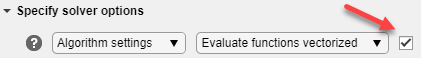## Vectorize the Fitness Function

### Vectorize for Speed

The genetic algorithm usually runs faster if you vectorize the fitness function. This means that the genetic algorithm only calls the fitness function once, but expects the fitness function to compute the fitness for all individuals in the current population at once. To vectorize the fitness function,

• Write the file that computes the function so that it accepts a matrix with arbitrarily many rows, corresponding to the individuals in the population. For example, to vectorize the function

`$f\left({x}_{1},{x}_{2}\right)={x}_{1}^{2}-2{x}_{1}{x}_{2}+6{x}_{1}+{x}_{2}^{2}-6{x}_{2}$`

write the file using the following code:

`z =x(:,1).^2 - 2*x(:,1).*x(:,2) + 6*x(:,1) + x(:,2).^2 - 6*x(:,2);`

The colon in the first entry of `x` indicates all the rows of `x`, so that `x(:, 1)` is a vector. The `.^` and `.*` operators perform elementwise operations on the vectors.

• At the command line, set the `UseVectorized` option to `true` using `optimoptions`.

• In the Optimize Live Editor task, ensure that the Algorithm settings > Evaluate functions vectorized setting has a check mark.Note

The fitness function, and any nonlinear constraint function, must accept an arbitrary number of rows to use the Vectorize option. `ga` sometimes evaluates a single row even during a vectorized calculation.

The following comparison, run at the command line, shows the improvement in speed with vectorization.

```options = optimoptions('ga','PopulationSize',2000); tic;ga(@rastriginsfcn,20,[],[],[],[],[],[],[],options);toc Optimization terminated: maximum number of generations exceeded. Elapsed time is 12.054973 seconds. options = optimoptions(options,'UseVectorized',true); tic; ga(@rastriginsfcn,20,[],[],[],[],[],[],[],options); toc```
```Optimization terminated: maximum number of generations exceeded. Elapsed time is 1.860655 seconds.```

### Vectorized Constraints

If there are nonlinear constraints, the objective function and the nonlinear constraints all need to be vectorized in order for the algorithm to compute in a vectorized manner.

Vectorize the Objective and Constraint Functions contains an example of how to vectorize both for the solver `patternsearch`. The syntax is nearly identical for `ga`. The only difference is that `patternsearch` can have its patterns appear as either row or column vectors; the corresponding vectors for `ga` are the population vectors, which are always rows.

Watch now